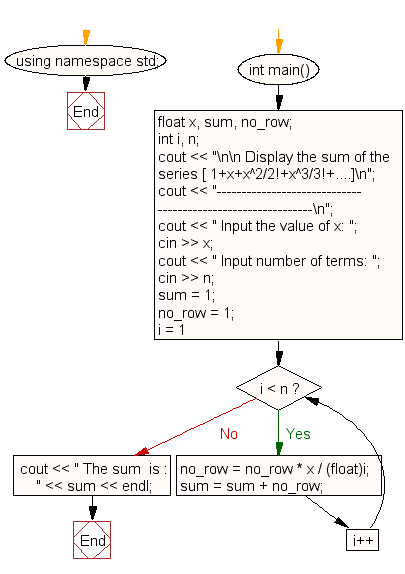﻿ C++ : Display the sum of the series 1+x+x^2/2!+x^3/3!+....# C++ Exercises: Display the sum of the series 1+x+x^2/2!+x^3/3!+....

## C++ For Loop: Exercise-24 with Solution

Write a program in C++ to display the sum of the series [ 1+x+x^2/2!+x^3/3!+....].

Sample Solution:

C++ Code :

``````#include <iostream>
using namespace std;

int main()
{
float x, sum, no_row;
int i, n;
cout << "\n\n Display the sum of the series [ 1+x+x^2/2!+x^3/3!+....]\n";
cout << "------------------------------------------------------------\n";
cout << " Input the value of x: ";
cin >> x;
cout << " Input number of terms: ";
cin >> n;
sum = 1;
no_row = 1;
for (i = 1; i < n; i++)
{
no_row = no_row * x / (float)i;
sum = sum + no_row;
}
cout << " The sum  is : " << sum << endl;
}
``````

Sample Output:

``` Display the sum of the series [ 1+x+x^2/2!+x^3/3!+....]
------------------------------------------------------------
Input the value of x: 3
Input number of terms: 5
The sum  is : 16.375
```

Flowchart:C++ Code Editor:

Contribute your code and comments through Disqus.

What is the difficulty level of this exercise?

﻿

## C++ Programming: Tips of the Day

What is a smart pointer and when should I use one?

This answer is rather old, and so describes what was 'good' at the time, which was smart pointers provided by the Boost library. Since C++11, the standard library has provided sufficient smart pointers types, and so you should favour the use of std::unique_ptr, std::shared_ptr and std::weak_ptr.

There was also std::auto_ptr. It was very much like a scoped pointer, except that it also had the "special" dangerous ability to be copied - which also unexpectedly transfers ownership.

It was deprecated in C++11 and removed in C++17, so you shouldn't use it.

```std::auto_ptr<MyObject> p1 (new MyObject());
std::auto_ptr<MyObject> p2 = p1; // Copy and transfer ownership.
// p1 gets set to empty!
p2->DoSomething(); // Works.
p1->DoSomething(); // Oh oh. Hopefully raises some NULL pointer exception.
```

Ref : https://bit.ly/3mc9GHE